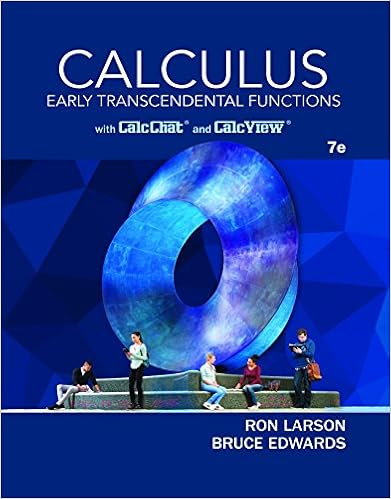# 15 16 1 point let f x x 2 8 x a find the slope of the

• Homework Help
• zhrngx68
• 5

This preview shows page 3 - 5 out of 5 pages.

##### We have textbook solutions for you!
The document you are viewing contains questions related to this textbook.The document you are viewing contains questions related to this textbook.
Chapter 5 / Exercise 65
Calculus: Early Transcendental Functions
Edwards/LarsonExpert Verified
-1/5
(B) Find the slope of the secant line joining(6,f(6))and
17.(1 point) a) The rectangles in the graph below illustrate a?
3
##### We have textbook solutions for you!
The document you are viewing contains questions related to this textbook.The document you are viewing contains questions related to this textbook.
Chapter 5 / Exercise 65
Calculus: Early Transcendental Functions
Edwards/LarsonExpert Verified
b) The rectangles in the graph below illustrate a?
Right endpoint Riemann sum fory=x211on[3,7]Solution:Solution:a) This is a left endpoint Riemann sum. Its value is:f(3)·0.5+f(3.5)·0.5+···+f(6.5)·0.5
•••